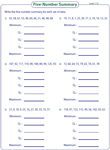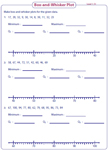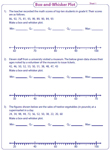1. Worksheets>
2. Math>
3. Statistics>
4. Box and Whisker Plot

# Box-and-Whisker Plot Worksheets

Box-and-whisker plot worksheets have skills to find the five-number summary, to make plots, to read and interpret the box-and-whisker plots, to find the quartiles, range, inter-quartile range and outliers. Word problems are also included.

Five-number summary

Write the five-number summary (first quartile, second quartile, third quartile, maximum and minimum) for the given sets of data.Level 1:

Level 2:

Make a box-and-whisker plot: Standard

Three sets of data are given in each worksheet. From the given data find the five-number summary and make a box-and-whisker plot.Level 1:

Level 2:

Make a box-and-whisker plot: Word problems

Read the given data carefully and determine the five-number summary to make box-and-whisker plots. Each worksheet has three problems.Read the scenarios and interpret the stem-and-leaf plot to answer the word problems based on the five-number summary.These worksheets have exclusive word problems to find the five-number summary, range and inter-quartile range.Make and interpret

For the given data, make a box-and-whisker plot. Interpret the data to find Q1, Q2, Q3, maximum and minimum values.Finding the outliers

Each worksheet has eight problems given. Find the outliers by computing the quartiles and the inter-quartile range.Develop skills with the entire collection of worksheets on box-and-whisker plot.

Related Worksheets